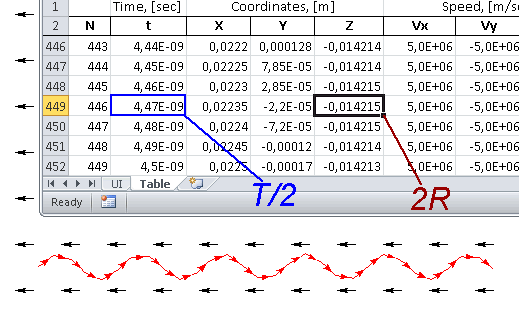QuickField

A new approach to field modelling

Main >> >> Charged particle trajectory in the uniform static magnetic field. Case: plane-parallel

# Charged particle trajectory in the uniform static magnetic field. Case: plane-parallel.

QuickField simulation example

Problem Type
Plane-parallel problem of DC magnetics.

Geometry

Given
Relative permeability of vacuum μ = 1;
External field flux density Bx = -4 mT.
Charge (electron) q = -1.602e-19 C;
Mass (electron) m = 9.109e-31 kg;
Initial velocity vx=vy=5e6 m/s; vz = 0 m/s.
Emitter position (0; 0; 0).

Calculate charged particle trajectory in magnetic field neglecting relativistic effects.

Solution
The analytical solution gives spiral trajectory.
Radius in YZ-plane RYZ = vy / Bx * m/q [m].
Period T = 2π / Bx * m/q [s].
Lorentz force Fz = q*vy*Bx [N].

To calculate the particle trajectory in QuickField free tool TrajectoryTracer is used.

Results

Analytical solution:
Radius in YZ-plane RYZ = (5e6/0.004) * (9.109e-31/1.602e-19) = 0.00711 m.
Period T = (2*3.142/0.04) * (9.109e-31/1.602e-19) = 8.93e-9 s.
Lorentz force Fz = 1.602e-19 * 5e6 * 0.04 = 3.20e-15 N.

TrajectoryTracer tool:
Radius in YZ-plane RYZ = 0.14215/2 = 0.00711 m.
Period T = 4.47e-9*2 = 8.94e-9 s.
Lorentz force Fz = 3.20e-15 N.